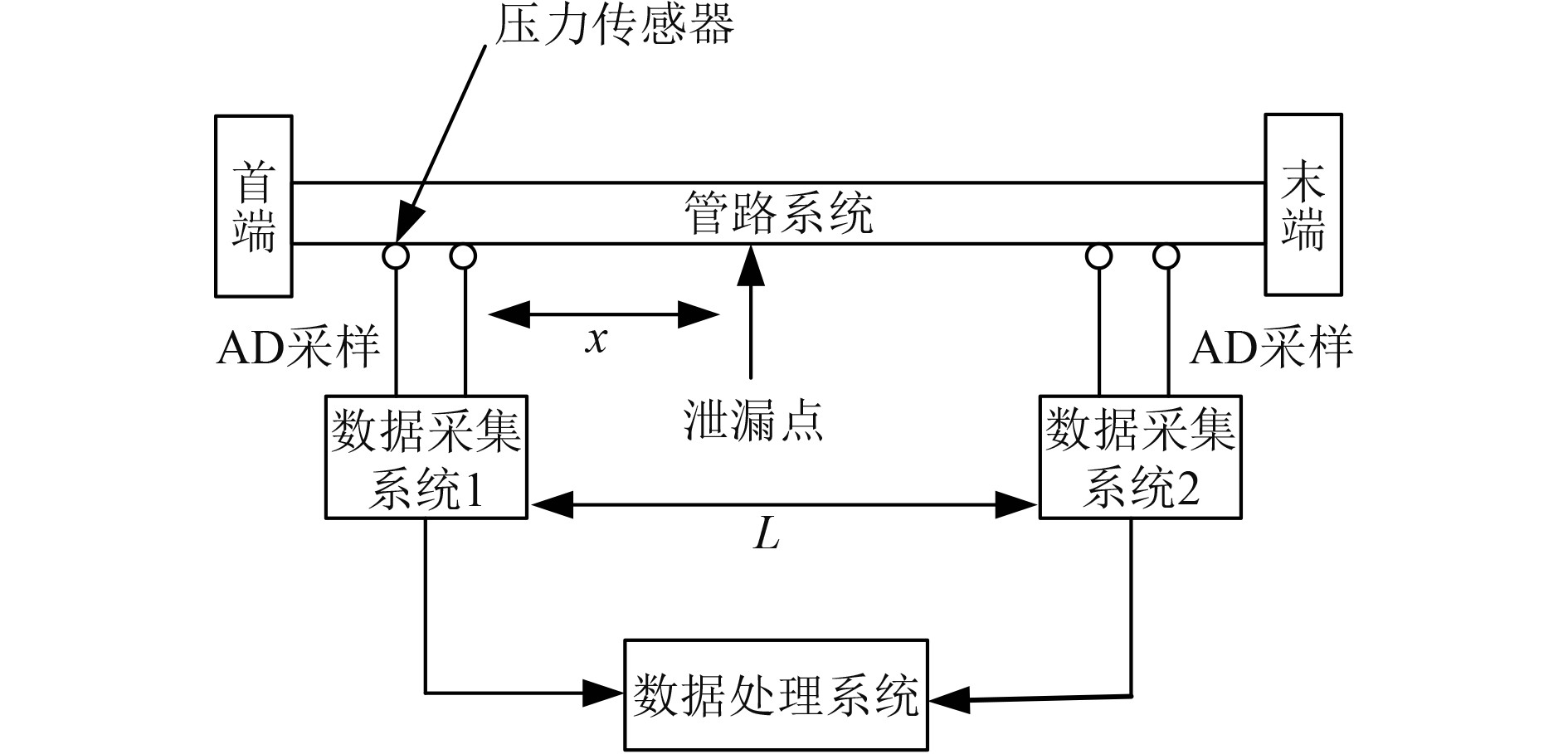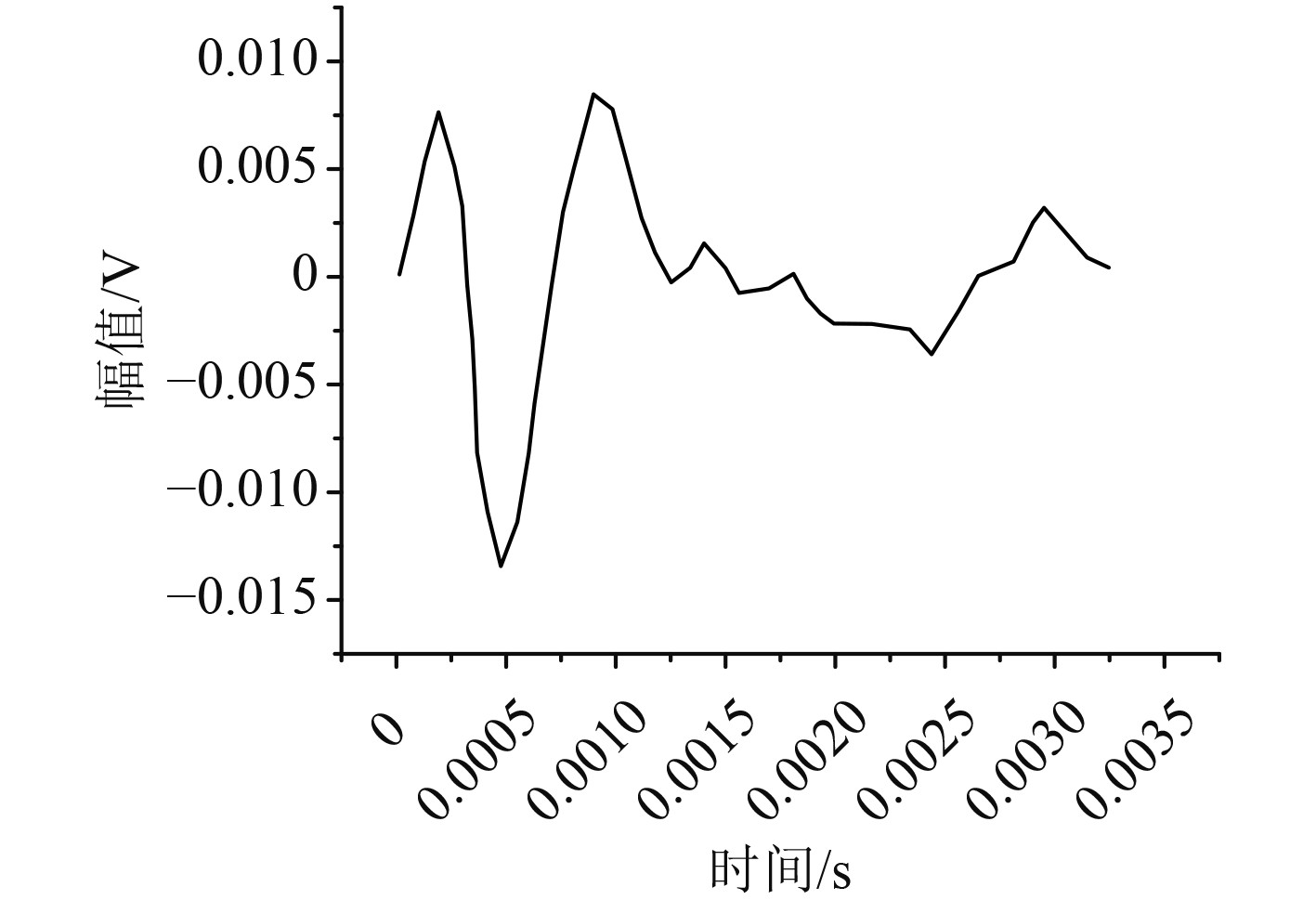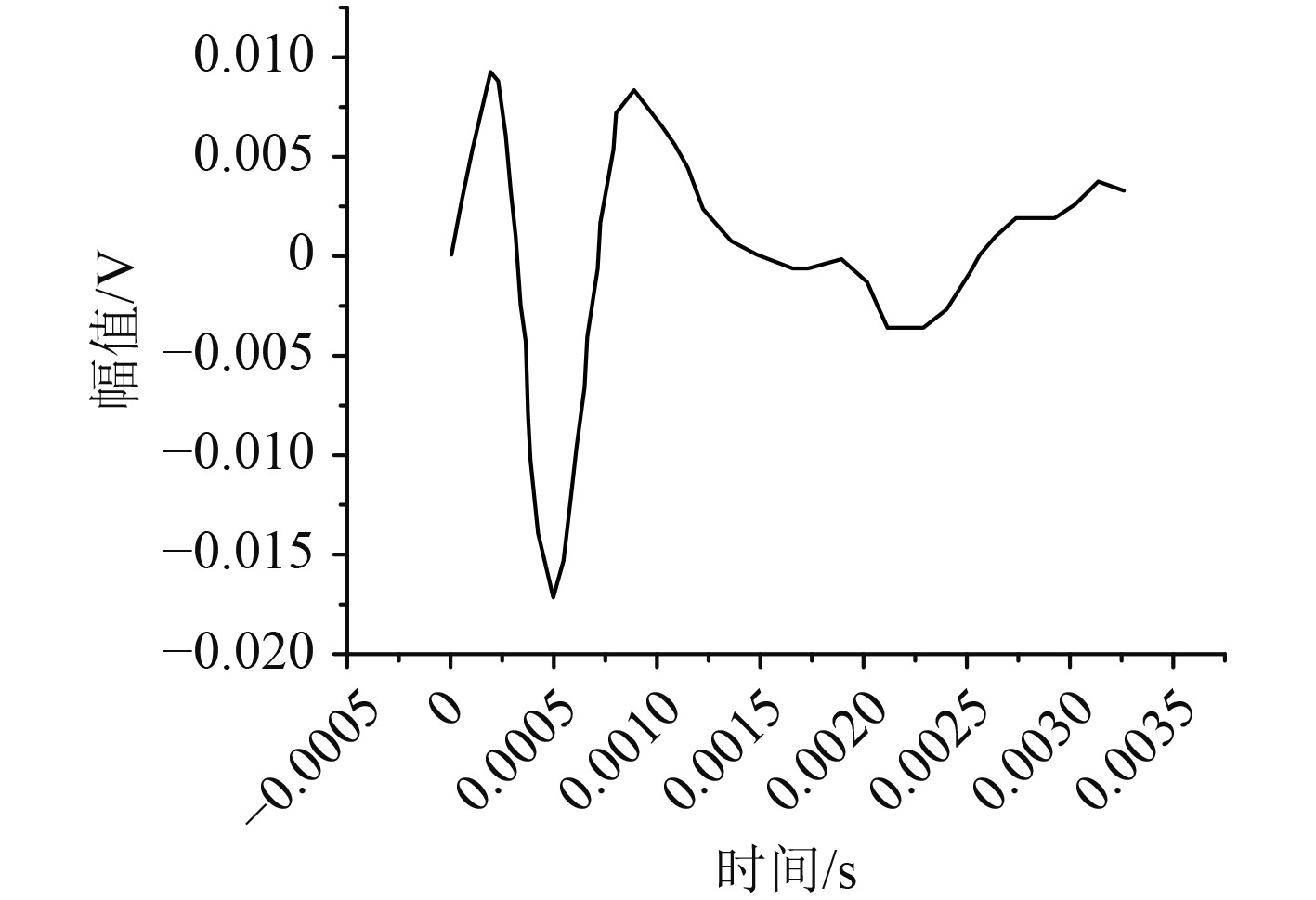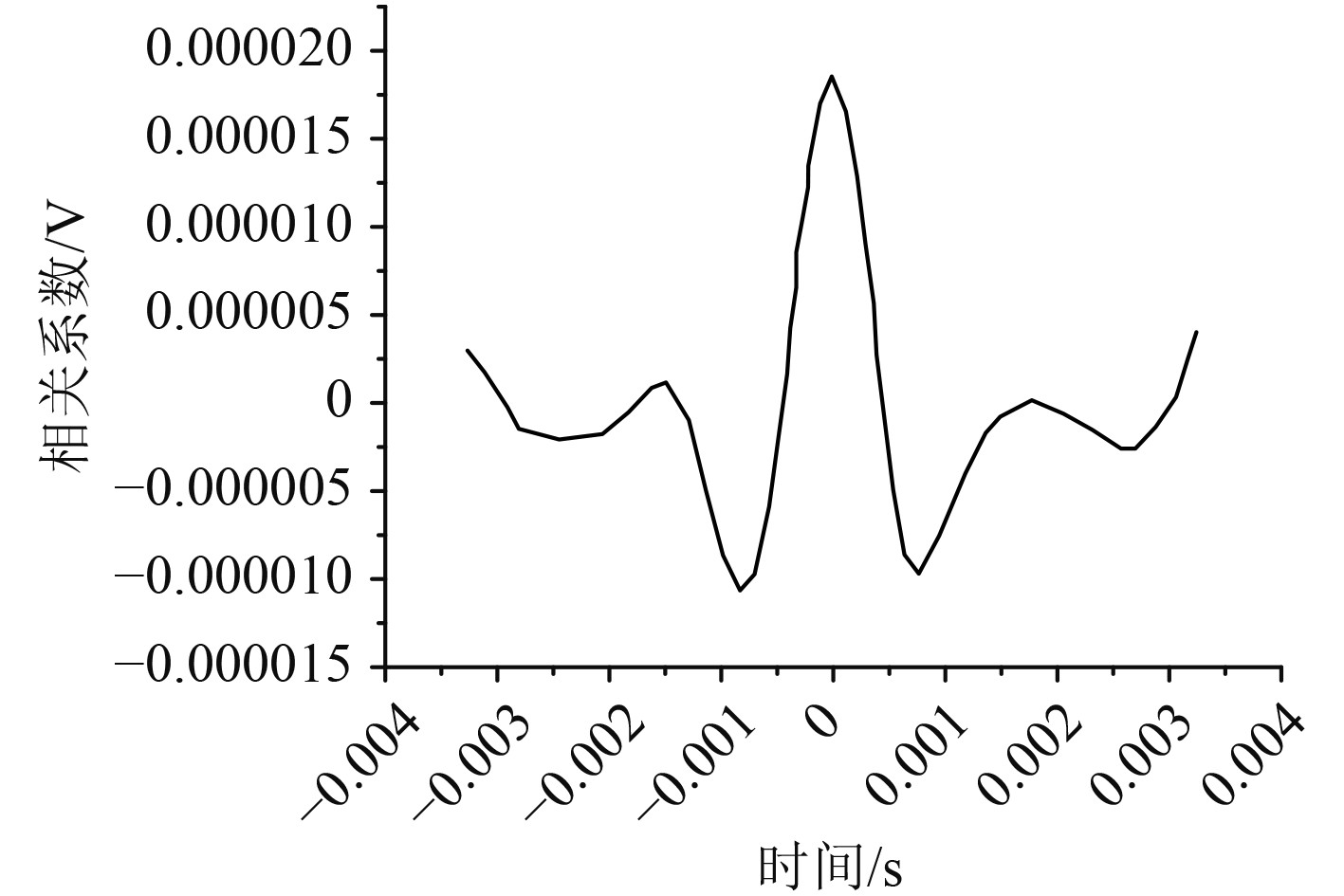﻿ 船舶管路系统液体泄漏监测仪的设计与开发
 舰船科学技术2022, Vol. 44Issue (8): 118-121    DOI: 10.3404/j.issn.1672-7649.2022.08.024PDF

1. 内蒙古农业大学 计算机技术与信息管理系，内蒙古 包头 014109;
2. 中国航天科工六院601所，内蒙古 呼和浩特 010076

Design and development of liquid leakage monitor for ship pipeline system
GAO Hong-wei1, LI Ming1, YIN Mei-xiu2
1. Computer Technology and Information Managemen, Inner Mongolia Agricultural University Department, Baotou 014109, China;
2. The 601st Institute, the 6th Academy, China Aerospace Science and Industry Corporation, Hohhot 010076, China
Abstract: This paper summarizes the classification of ship pipeline system, including inert gas pipeline system, fire safety pipeline system and liquid level telemetry pipeline system, and constructs the mathematical model of ship pipeline system layout; The flow field characteristics of ship pipeline are analyzed. Based on the flow field characteristics of pipeline, a ship pipeline transmission model is proposed; The load wave caused by pipeline damage and leakage of ship pipeline system is studied. According to the load wave theory, the liquid leakage monitor of ship pipeline system is designed, the pipeline damage and leakage point is tested according to the liquid leakage monitor, and the collected pressure change curve is analyzed.
Key words: ship     piping system     liquid leakage     monitor
0 引　言

1 船舶管路系统 1.1 船舶管路系统分类

 ${d_1} = 25 + 2.16\sqrt {C\left( {B + D} \right)} \text{。}$ (1)

 $d = \sqrt 2 {d_1}\text{，}$ (2)

 $Q = 5.75 \times {d^2}/{10^3}\text{，}$ (3)

 ${Q_f} \geqslant 1.25 \times Q\text{。}$ (4)

 $Q = \frac{{V \times 5}}{K} \times C\text{。}$ (5)

 $N = \frac{{{L_1} - \sqrt {{R^2} - \left( {\dfrac{{{A^2}}}{2}} \right)} }}{{\sqrt {{R^2} - \left( {\dfrac{{{B^2}}}{2}} \right)} }} + 2 \text{。}$ (6)

1.2 船舶管路系统布局数学模型

 $\min f\left( T \right) = \left[ {{f_1}\left( T \right),{f_2}\left( T \right),...,{f_m}\left( T \right)} \right]\text{，}$ (7)

 ${g_i}\left( T \right) = 0,i = 1,2\cdots , q \text{。}$ (8)

 ${O_G} = \displaystyle\bigcup_{i = 1}^k {O_i} = {O_1} \cup {O_2} \cup {O_3} \cup \cdots \cup {O_k}\text{。}$ (9)

2 船舶管路系统模型分析 2.1 船舶管路流场特性分析

 $\left\{ {\begin{array}{*{20}{c}} {x = x\left( {a,b,c,t} \right)} \text{，}\\ {y = y\left( {a,b,c,t} \right)} \text{，}\\ {z = z\left( {a,b,c,t} \right)} \text{。} \end{array}} \right.$ (10)

 ${h_f} = \sum {{h_l} + \sum {{h_m}} }\text{。}$ (11)

 ${v_1}{A_1} = {v_2}{A_2}\text{。}$ (12)

2.2 船舶管路传输模型

 $\frac{{\partial v}}{{\partial t}} + v\frac{{\partial v}}{{\partial x}} + \frac{1}{\rho }\frac{{\partial H}}{{\partial x}} + g\sin \alpha + \frac{\lambda }{{2D}}{v^2} = 0\text{。}$ (13)

 $\frac{{\partial H}}{{\partial t}} + v\frac{{\partial H}}{{\partial x}} + \rho {a^2}\frac{{\partial v}}{{\partial x}} = 0\text{。}$ (14)

 $\left\{ {\begin{array}{l} {\dfrac{{\partial H}}{{\partial x}} + v\dfrac{{\partial H}}{{\partial x}} + \rho {a^2}\dfrac{{\partial v}}{{\partial x}} = 0} \text{，}\\ {g{H_x} + V{V_x} + {V_t} + \dfrac{f}{{2D}}V\left| V \right| = 0} \text{。} \end{array}} \right.$ (15)
3 船舶管路系统液体泄漏监测仪设计图 1 船舶管路系统液体泄漏监测仪 Fig. 1 Liquid leakage monitor for ship pipeline system

 $x = \frac{{L + a\Delta t}}{2}\text{。}$ (16)

 $a = \sqrt {\frac{{K/\rho }}{{1 + \dfrac{K}{E} \times \dfrac{D}{e} \times C}}}\text{。}$ (17)
4 船舶管路系统液体泄漏信号测量图 2 数据采集系统1采集到的信号 Fig. 2 Signal collected by data acquisition system 1图 3 数据采集系统2采集到的信号 Fig. 3 Signals collected by data acquisition system 2图 4 数据采集系统1和系统2采集信号间的互相关系数 Fig. 4 Cross correlation coefficient between signals collected by data acquisition system 1 and 2
5 结　语

  王德选, 陈秀玲, 陈红. 协同进化算法在船舶管路布局优化的应用[J]. 舰船科学技术, 2021(43): 49-51.  阳子轩, 范世东, 熊庭. 船舶管路泄漏时信号组成分析及预处理研究[J]. 武汉理工大学学报, 2011(35): 313-316.  陈丕智, 徐本川, 刘忠林, 等. 船舶管路设计中连接件和多芯管的应用[J]. 船海工程, 2013(42): 190-193.  张宇, 张硕, 王亚伟, 等. 船舶管路泄漏诊断技术综述[J]. 舰船电子工程, 2020(40): 19-23.  吴绍科, 付立东, 张跃文, 等. 船舶管路系统泄漏定位实验研究[J]. 舰船科学技术, 2017(39): 164-167.  董宗然, 林焰. 船舶布管系统的结构设计及自动化算法[J]. 计算机集成制造系统, 2016(22): 714-727.